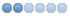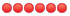# Symmetry of a transition density matrix

• leos
• Topic Author
• Offline
• Junior Member
•5 years 4 months ago #259 by leos
Dear Mihály,

I have a question about the symmetry of the transition density matrices.
In the CCDENSITIES file one can see that at least the D(I,J) block of the matrix is symmetric with respect to interchange of I and J.
Besides, one can see only the D(A,I) block in the file while the D(I,A) block is missed.
Does it mean that D(I,A)=D(A,I) similar to D(I,J)?
I cannot see why this is so for the transition density matrix case.
In the simplest case of the 1s->2s transition in the hydrogen atom one can expect that only the D(1,2) term should not be zero (and equal to 1.0), while D(2,1) should be zero.
In the test calculation D(1,2)=0.5 and I suppose that D(2,1)=0.5?
Thus, does MRCC code use some symmetrization of the transition density matrix?

Also I have a question about the two-electron density matrices.
Am I right that you use the G(1,2;1'2') particle numbering convention and not the G(1,1';2,2') ?

In the two electron density matrices some blocks are missed.
Am I right that it is supposed that, e.g. G(IA,KJ)=G(IJ,KA)?

Thank you,
Leonid Skripnikov.

• kallay
• Offline
•• Mihaly Kallay
5 years 4 months ago #260 by kallay
Dear Leonid,
For transition densities the CCDENSITIES file is not correct, do not use it. At gradient calculations the normal density matrix is written to the file utilizing the symmetry of matrix. At transition moment calculations the file is also written but not used. I will fix this.

Best regards,
Mihaly Kallay

• leos
• Topic Author
• Offline
• Junior Member
•5 years 4 months ago - 5 years 4 months ago #261 by leos
Dear Mihály,
Thank you, it would be very great to have such a unique option to calculate one-electron and two-electron transition density matrices.

Leonid Skripnikov.
Last edit: 5 years 4 months ago by leos.

• leos
• Topic Author
• Offline
• Junior Member
•4 years 10 months ago #295 by leos
Dear Mihály,
Is it possible to generate the transition density matrix CCDENSITIES file with a correct symmetry (i.e. not with the symmetry of the diagonal DM) in the latest (2016-07-15) version of MRCC?

Also it seems that in the new version of MRCC the "dens=5" option works slightly incorrect in the case of Dirac calculations: if I set dens=5, I get not only 1-electron but also 2-electron transition density matrix, i.e. "dens=5" option works as "dens=6" option. This is not good for some cases because it tooks a lot of time and disk space to compute (sometimes unnecessery) 2-electron DM.

Thank you,
Leonid Skripnikov.

• kallay
• Offline
•• Mihaly Kallay
4 years 10 months ago #296 by kallay
Dear Leonid,
The transition density in the CCDENSITIES is still not correct, sorry.
But if you use Dirac, you can, in principle, pass over any one-electron property integrals to mrcc, and these will be contracted with the transition density matrix. There may be problems if your integral list has different symmetries than the dipole integrals, but it is probably easier to fix these than to print out the correct transition densities into the CCDENSITIES file.
To avoid the calculation of two-particle density matrices, edit dmrcc.f and remove the
if(mod(dens,2).eq.1) dens=dens+1
line from subroutine write56.

Best regards,
Mihaly Kallay
The following user(s) said Thank You: leos

• leos
• Topic Author
• Offline
• Junior Member
•4 years 10 months ago - 4 years 10 months ago #297 by leos
Dear Mihály,

Thank you!
Last edit: 4 years 10 months ago by leos.